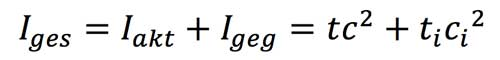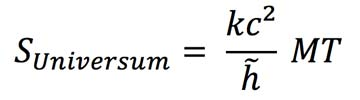# Presentation of the book

## Do you want to understand the basic structure of space?

If the physical constants of the speed of light, the quantum of action and elementary time possess the elementary value of 1, all the essential physical quantities derived from them with their formulas also add up to 1. This postulate leads to a universal elementary unit system, comparable to the Planck units.

## Are you fascinated by numbers?

The sum of least squares π2/6, the isentropic exponent of space (κ) and the square of the prime number 13 give the enigmatic fine structure constant (α).

## Do you love to calculate?

The expansion of space follows clear physical rules. It is an isentropic expansion in which mass decreases.

## Do you like physical formulas?

The information of the future is formed by the information of the past and of the presentThe product of the mass and the age of space remains constant during its expansion. M stands for the mass of space today while T represents the age of space, i.e. 13.8 billion years.## What is the cause of gravitation?

A universal mass disintegration , which forms time and space, affects every object in the universe.

When dipole decay in the masses arise with time magnetic monopoles, which exert a magnetostatic force on each other.